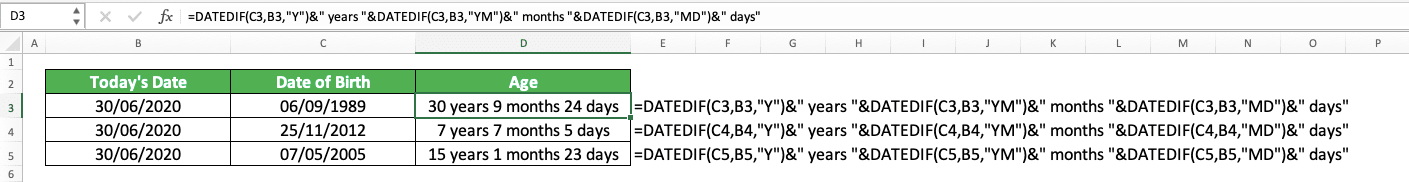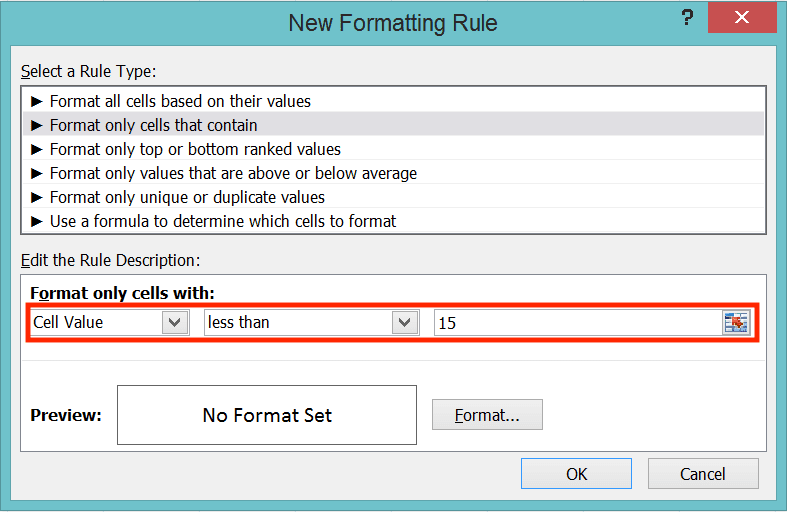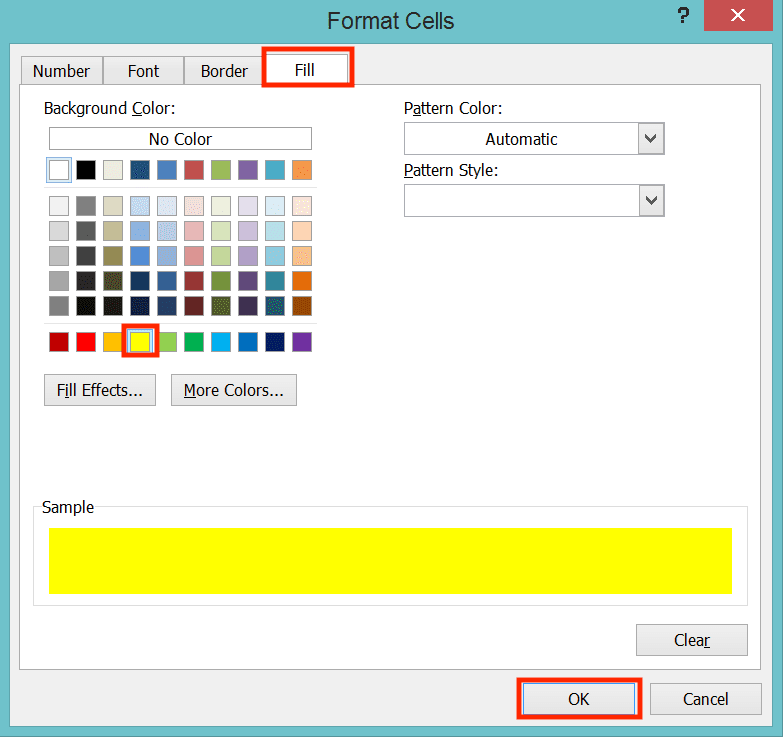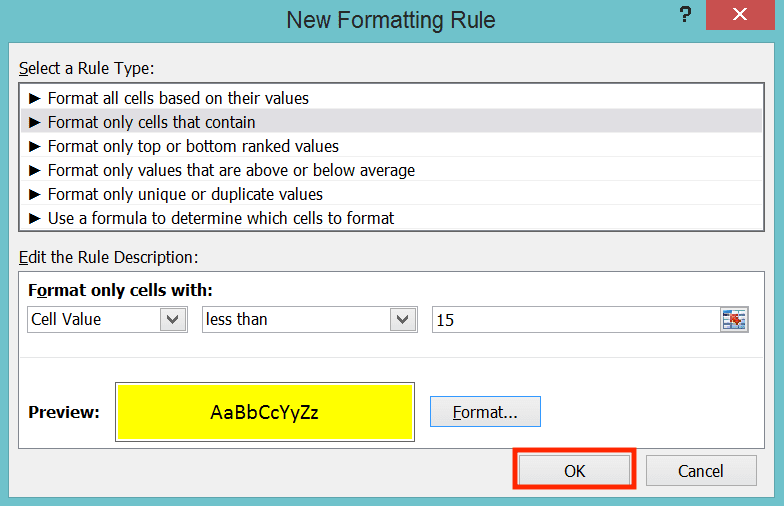How to Calculate Age in Excel - Compute Expert

# How to Calculate Age in Excel

Home >> Excel Tutorials from Compute Expert >> Excel Tips and Trick >> How to Calculate Age in Excel

In this tutorial, you will learn how to calculate age in excel completely. There are many methods you can use depending on what kind of age result you want to get.

Age is a variable we sometimes need to find when we process our data in excel. When you have mastered how to calculate it, you can do your excel work more optimally.

What are the methods to calculate age in excel and how to implement them? Join us in discussing them in this tutorial!

Disclaimer: This post may contain affiliate links from which we earn commission from qualifying purchases/actions at no additional cost for you. Learn more

## How to Calculate Age in Excel 1: Manual Formula Writing

First, let’s discuss the basic methods to calculate age in excel before we discuss how to get a specific age result.

There are three basic methods you can use to calculate age in excel we will discuss here. The first one is by using manual formula writing.

Age calculation is basically a subtraction process. You subtract a particular date with the Date of Birth (DoB) to get the age number.

As such, we can write a manual subtraction formula in our cell to get the age number we want like this.

= ( date_from_which_we_calculate_the_age - date_of_birth ) / 365

You can use cell coordinates or direct typing to input the dates in the subtraction formula there. If you type them directly, then you should use quotes on your dates (e.g. “12/2/2001” for 12 February 2021).

We divide the days result of the subtraction with 365 as that is the number of days in a normal year. From the formula writing, we will get a decimal number that represents the age we want to get.

Here is the implementation example of the formula writing in excel.For today’s dates in the example, we can use the TODAY formulas to get the dates fast. We can also input the formula directly into our manual formula writing if we want. TODAY will keep on updating today’s date whenever you open or edit its worksheet.

Subtract dates manually and divide the result with 365. We should get our age number from there.

## How to Calculate Age in Excel 2: YEARFRAC

Another method to calculate age in excel is by using the YEARFRAC formula. From YEARFRAC, you can get the difference of two dates in the form of a decimal number and a year unit. The result is quite similar to the manual formula writing we discussed just now.

Here is the general writing form of YEARFRAC in excel to calculate age.

= YEARFRAC ( date_of_birth , date_from_which_we_calculate_the_age , [ calculation_basis ] )

You can swap the input position of the birth date and age basis date and still get the same result. For the calculation basis, there are five input options you can choose from. Those options differ in their number of days basis in a month and in a year for the dates subtraction calculation.

Here is a table that summarizes the input options and their calculation basis.

Input OptionNumber of Days in a Month for the Calculation BasisNumber of Days in a Year for the Calculation Basis
030 (US basis)360
1actualactual
2actual360
3actual365
430 (Europe basis)360

The calculation basis is important because it will determine the age value you will get from YEARFRAC. A fewer number of days in a month or in a year means a larger age result for you (because we will round the number of days difference between our dates to larger year and month numbers).

The word “actual” there means excel will see the actual number of days in the years and months between our dates. If, for example, there is 2016 between our dates, then the number of days for that year will be 366 days. That is because 2016 is a leap year.

The difference between US basis and European basis there is the way they handle the last day of a month. For the US basis, if the first date input is on its month’s last day, then the day will become 30. If the second date input is on its month’s last day, then excel will take a look at the first date.

If the first date’s day is less than 30, the second date will become the first day of its next month. If the first date’s day is 30 or 31, then the second date’s day becomes 30.

For the European basis, if the day of the first date and/or second date is 31, it will become 30.

This YEARFRAC’s calculation basis input, by the way, is optional. If you don’t input anything there, then excel will assume your input is 0.

Here is the YEARFRAC implementation example in excel to calculate age.If you use a different calculation basis, then the age calculation results will change.Therefore, if you use YEARFRAC to calculate age in excel, pay attention to the calculation basis you use!

## How to Calculate Age in Excel 3: DATEDIF

The last basic age calculation method we will give in this tutorial is by using the excel secret formula, DATEDIF. We call it a secret formula because excel won’t show suggestions when you try to type this formula in your cell (give it a try if you haven’t used this formula before).

DATEDIF is a formula that specializes itself in finding the days, months, and/or years difference between two dates. How to write this formula to calculate age in excel? Well, here is the general writing form.

= DATEDIF ( date_of_birth , date_from_which_we_calculate_the_age , calculation_unit )

The first two inputs seem clear enough as you input the dates to calculate your age number. The calculation unit input there, however, determines the kind of result you will get from DATEDIF.

Here is a table that summarizes the calculation unit input options and what they will calculate for you. You need to input this calculation unit with quotes (“”) in your DATEDIF (unless you input through a cell coordinate, of course).

Yyears
Mmonthsconsiders the number of years when doing the calculation
Ddaysconsiders the number of years and months when doing the calculation
MDdaysdoesn’t consider the number of years and months when doing the calculation
YMmonthsdoesn’t consider the number of years when doing the calculation
YDdaysconsider the number of months and doesn’t consider the number of years when doing the calculation

If you use DATEDIF for your age calculation, then you will get a whole number result. That result can be the difference of years, months, or days depending on the calculation unit you input.

As we calculate age here, we most probably calculate the difference of years, in which we use the “Y” calculation unit. That is unless you want to know the difference between months and days too in the age.

In the additional note column in the table, you see the words “consider” and doesn’t consider”. To understand this, let’s say we use DATEDIF to calculate the months’ difference between two dates.

If we consider the years (using the “M” calculation unit), then we will convert the years to months and subtract them too to get our result (e.g. the months’ difference calculation result between 10 August 2010 and 28 November 2011 becomes 15). If we don’t consider the years (using the “YM” calculation unit), then we will just subtract the months (e.g. the months’ difference calculation result between 10 August 2010 and 28 November 2011 becomes 3).

That is for the discussion about how to use DATEDIF generally. Here is an implementation example of the formula to calculate age in excel.As you can see there, we input the date of birth, today’s date, and “Y” calculation unit to our DATEDIF. As a result, we get our age in whole numbers and a year unit.

If you need a clean age calculation result by using only one formula, then you should use DATEDIF.

## How to Calculate Age in Excel from Date of Birth (DoB) for Its Year Number with Decimals Remainder

If you need your age number in its decimal form, then you should use manual formula writing or YEARFRAC. As you can see from our previous discussion, those two methods will give you your age number in decimals.

Below, you can see how we calculate our dates again with manual formula writing and YEARFRAC.Don’t forget to use the right calculation basis to get the correct age for you if you use YEARFRAC.

## How to Calculate Age in Excel from Date of Birth (DoB) for Its Full-Year Number

If you need a whole number for your age calculation result, then you can use DATEDIF with the “Y” calculation unit.

Alternatively, you can also apply the INT formula to your manual formula writing/YEARFRAC if you want to use them instead. INT is an excel formula that will round down a decimal number to its nearest whole number.

Here are the general writing forms of the three methods if you want a full-year number for your age.

DATEDIF

= DATEDIF ( date_of_birth , date_from_which_we_calculate_the_age , “Y” )

Manual formula writing with INT

= INT ( ( date_from_which_we_calculate_the_age - date_of_birth ) / 365 )

YEARFRAC with INT

= INT ( YEARFRAC ( date_of_birth , date_from_which_we_calculate_the_age , [ calculation_basis ] ) )

Using INT with our manual formula writing/YEARFRAC is easy as you can see above. We just need to input the formula we use into our INT. By doing that, we will get our age calculation result in its year unit and whole number form.

Here is an implementation example of our three age calculation methods to get the result we want.As you can see there, we get our age calculation results in their full-year numbers using the three methods. For manual formula writing and YEARFRAC methods, we apply INT to their results. For DATEDIF, we input a “Y” calculation unit.

## How to Calculate Age in Excel from Date of Birth (DoB) in Years, Months, and Days

If you need to get your age number from its year to its day number, then you should use multiple DATEDIFs. We can concatenate those DATEDIFs using the ampersand symbol (&) so we will get the age calculation result we want.

Here is the combination of those DATEDIFs and ampersand symbols in one writing form for our calculation process.

= DATEDIF ( date_of_birth , date_from_which_we_calculate_the_age , “Y” ) & “ years “ & DATEDIF ( date_of_birth , date_from_which_we_calculate_the_age , “YM” ) & “ months “ & DATEDIF ( date_of_birth , date_from_which_we_calculate_the_age , “MD” ) & “ days “

By using this formula writing, we can get our age in year, month, and day numbers. We get them by inputting “Y”, “YM”, and “MD” as our DATEDIFs’ calculation units.

We can also add “years’, “months”, and “days” text there if we want as clear notes for the numbers. Don’t forget to add spaces in the quotes if you add those text so the result will look neat.

For better understanding, here is the implementation example of the formula writing to calculate age in excel.The formula writing is quite long as you can see in the example. However, the concept is simple as we only combine some DATEDIFs and text using ampersand symbols here.

We write our formula using the writing form we have discussed to calculate the age there. As a result, we get a complete age number in years, months, and days units!

## How to Calculate Age in Excel on a Specific Date

Need to calculate the age number at a date other than today’s date? You just need to change today’s date input in your age calculation formula writing to the date you want.

Here is the implementation example of that in excel using the three age calculation methods we have learned.You can see there how we use various dates as the basis of our age calculations. However, they aren’t a problem as we just need to input those dates in our formula writing.

Input the specific dates you want to use correctly in your formula and you should immediately get your age calculation results!

## How to Calculate Age in Excel in a Specific Year

What if you only have the year from which you want to base your age calculation in excel? For that, you may want to extract the year from your date of birth data first using the YEAR formula. After that, you subtract your year number with the year from the date of birth.

Here is the general writing form of the formulas you need to do that.

= year_from_which_we_calculate_the_age - YEAR ( date_of_birth )

Just need to input our date of birth to the YEAR formula and we will immediately get its year number.

Here is the implementation example of the formula writing in excel to calculate the age numbers.From this calculation, we will get our age calculation results in a whole number form and year unit.

## How to Calculate When a Person Will Reach a Specific Age in Excel

To know when will a person reach a specific age, just add the age number to the date of birth year. To do that in excel, get the year number of the date of birth first by using YEAR.

You need to also get the month and day numbers from your date of birth to create the specific age date. You can use the MONTH and DAY formulas for that. After that, combine all the information you have got using the DATE formula.

To summarize all of those, here is the formula writing form to get the date of a specific age in excel.

= DATE ( YEAR ( date_of_birth ) + specific_age_number , MONTH ( date_of_birth ) , DAY ( date_of_birth ) )

The YEAR, MONTH, and DAY formulas will extract the year, month, and day numbers from your date of birth for you. Meanwhile, the DATE formula will give you a date by using the numbers as its inputs.

For a better understanding of the concept, here is its implementation example.Using the combination of the formulas we use, we get the dates for the specific age numbers we need!

## How to Calculate Age in Excel When the Date of Birth (DoB) Year, Month, and Day Numbers Are in Different Cells

Your date of birth’s year, month, and day numbers are all in separate cells, not in one date? If that is the case, then you should join them first by using DATE before calculating your age number.

After joining the numbers, then you can use manual formula writing, YEARFRAC, or DATEDIF to calculate the age. You can place the DATE formula you use in a cell first or input it directly to your age calculation formula.

Here is the general writing form of DATE to combine your date of birth’s year, month, and day numbers.

= DATE ( date_of_birth_year , date_of_birth_month , date_of_birth_day )

And here is the implementation example of the concept. In the example, we put the DATE results in cells first and we use DATEDIF to calculate the ages.If you want to use the manual formula writing or YEARFRAC method, then the way to do it is similar. Just use DATE to combine your year, month, and day numbers first before inputting its result to your age calculation method.

## Highlight Ages Based on Their Age Range

Need to color code the age numbers in your cells based on what age range they fall into? You can do that by using the conditional formatting feature in excel.

Just set the rules to give the appropriate color for each age range you have and you should be good. To give you a better understanding of the method, let’s take a look at the example below.

Let’s say we have age numbers like in the screenshot below. We want to color-code their cells based on the guidance in the table on the right.How to do that? First, highlight all the cells where you want to apply the conditional formatting rules. Then, go to the Home tab, click on the Conditional Formatting button, and choose Manage Rules….In the dialog box that shows up, click New Rule….Set colors based on our age range in the dialog box that shows up. To do that, first, choose “Format only cells that contain” in the “Select a Rule Type” box.Then, in the part below the box, we set the colors. For the first color in the example (yellow for age numbers less than 15), we choose “Cell value” and “less than” in the dropdowns there. After that, we type 15 in the text box on its right.Below that is the part where we set the color for the age range we input. Click on the Format… button there.In the dialog box that shows up, you can choose the cell format you want for your age range. For the example, we go to the Fill tab there and choose the yellow color. After that, we click OK.Click OK after you finish setting the highlight format for the first age range.The rule you established will be there in the rule list of the cells’ conditional formatting. Repeat all the steps since we click the New Rule… button for all the age ranges you have. After you finish setting all the highlight formats for your age ranges, click OK.Excel will immediately highlight the cells containing your age numbers according to your rules. That is how you highlight ages based on their age ranges in excel!## Exercise

After learning how to calculate age in excel completely, now is the time to do an exercise. You should do this exercise so you can understand what you have just learned more practically.

### Questions:

1. How many years old is each of the people? We only need the full-year numbers in here. The calculation is today’s day (use TODAY in your age calculation formula)!
2. How many years old is each of the people? We need the decimal of months and days in here. The calculation is today’s day (use TODAY in your age calculation formula)!
3. How many years old is each of the people? We need the number of years, months, and days in here. The basis for the calculation is 31 December 2020

If you want today’s date that doesn’t update itself every time, then you can paste value your TODAY formula. Alternatively, you can just press Ctrl + ; simultaneously to input today’s date in a cell.

Related tutorials you should learn:

Get updated excel info from Compute Expert by registering your email. It's free!